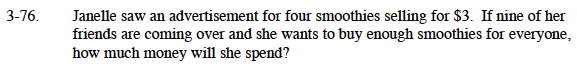### Home > MC1 > Chapter 3 > Lesson 3.3.2 > Problem3-76

3-76.First, calculate the cost of one smoothie.

Now that you know how much Janelle will need for 1 smoothie, the next step is to calculate how much money Janelle will need for 10 smoothies.

$\frac{3}{4} = 0.75$

$0.75 per smoothie ($0.75)(10) = ?